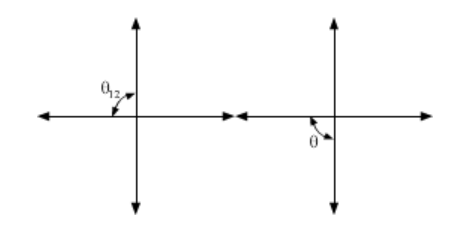# If sin θ=

Question:

If $\sin \theta=-\frac{4}{5}$ and $\theta$ lies in third quadrant, then the value of $\cos \frac{\theta}{2}$ is

(a) $\frac{1}{5}$

(b) $-\frac{1}{\sqrt{10}}$

(c) $-\frac{1}{\sqrt{5}}$

(d) $\frac{1}{\sqrt{10}}$

Solution:$\sin \theta=\frac{-4}{5}$

where $\theta$ lies in third quadrant.

since $\cos ^{2} \theta=1-\sin ^{2} \theta$

$=1-\left(\frac{-4}{5}\right)^{2}$

$=1-\frac{16}{25}$

$\cos ^{2} \theta=\frac{9}{25}$

i. e. $\cos \theta=\pm \frac{3}{5}$

since $\pi<\theta<\frac{3 \pi}{2}$

i. e. $\cos \theta=-\frac{3}{5}$

$\Rightarrow \frac{\pi}{2}<\frac{\theta}{2}<\frac{3 \pi}{4}$

lies in II quadrant using identity,

$\cos \frac{\theta}{2}=\pm \sqrt{\frac{1+\cos \theta}{2}}$

$=\pm \sqrt{\frac{1-\frac{3}{5}}{2}}$

$=\pm \sqrt{\frac{5-\frac{3}{5}}{2}}$

$=\pm \sqrt{\frac{2}{5} \times \frac{1}{2}}$

$=\pm \frac{1}{\sqrt{5}}$

since $\frac{\theta}{2}$ lies II quadrant $=\cos \frac{\theta}{2}=-\frac{1}{\sqrt{5}}$

Hence, the correct answer is option C.